Subscribe below
Oops! Something went wrong while submitting the form.

### ⛰️📈Show me the 5,436,563 ways to climb this mountain

At Traverse we perform micro-siting for wind farms by using IRR, NPV or LCOE as the target optimization variables. If you look at our landing page, it says we help project developers increase greenfield IRR by 0.5%. To fulfill this promise, we have to perform three key tasks.

• A. energy and wake modelling which covers revenue
• B. roads and foundation CAPEX optimization which covers costs and
• C. electrical array cable optimization which affects both revenue and cost.

These 3 topics are intertwined as every time you move a turbine it affects the optimality of all other turbines in a recursive cascade.

This article is a showcase on how we perform B, roads and foundation CAPEX optimization. In particular how we find the best ways to build roads up a mountain. We use two key algorithms in computer science: A* Search and Minimum Spanning Trees (MST) and scaled it to work on up to 500 turbines and a 100km by 100km boundary.

#### 0. Reference Wind Farm

The example wind farm we are working on today has 4 turbines, 1 access point and 2 substations in the Lingayen Gulf of the Philippines. It is situated in complex terrain for the sake of giving this example.

#### 1. The Optimal Path Between Two Turbines

Let’s look at the simplest case of one road connection between an access point and the very first turbine. What is the cheapest way to connect "Access Road" with Turbine 1 (T1)? The road specifications must fulfill:

• longitudinal slope must be between 10% to 14% - anything below 10% results in an unsealed road and between 10-14% results in a sealed road
• turning radii should not exceed 60m
• a road width of 6m
• sealed roads cost $150/m (we have seen up to$1,000/m) and unsealed roads cost $50/m • excavation for cut and fill costs between$5 to $10/m3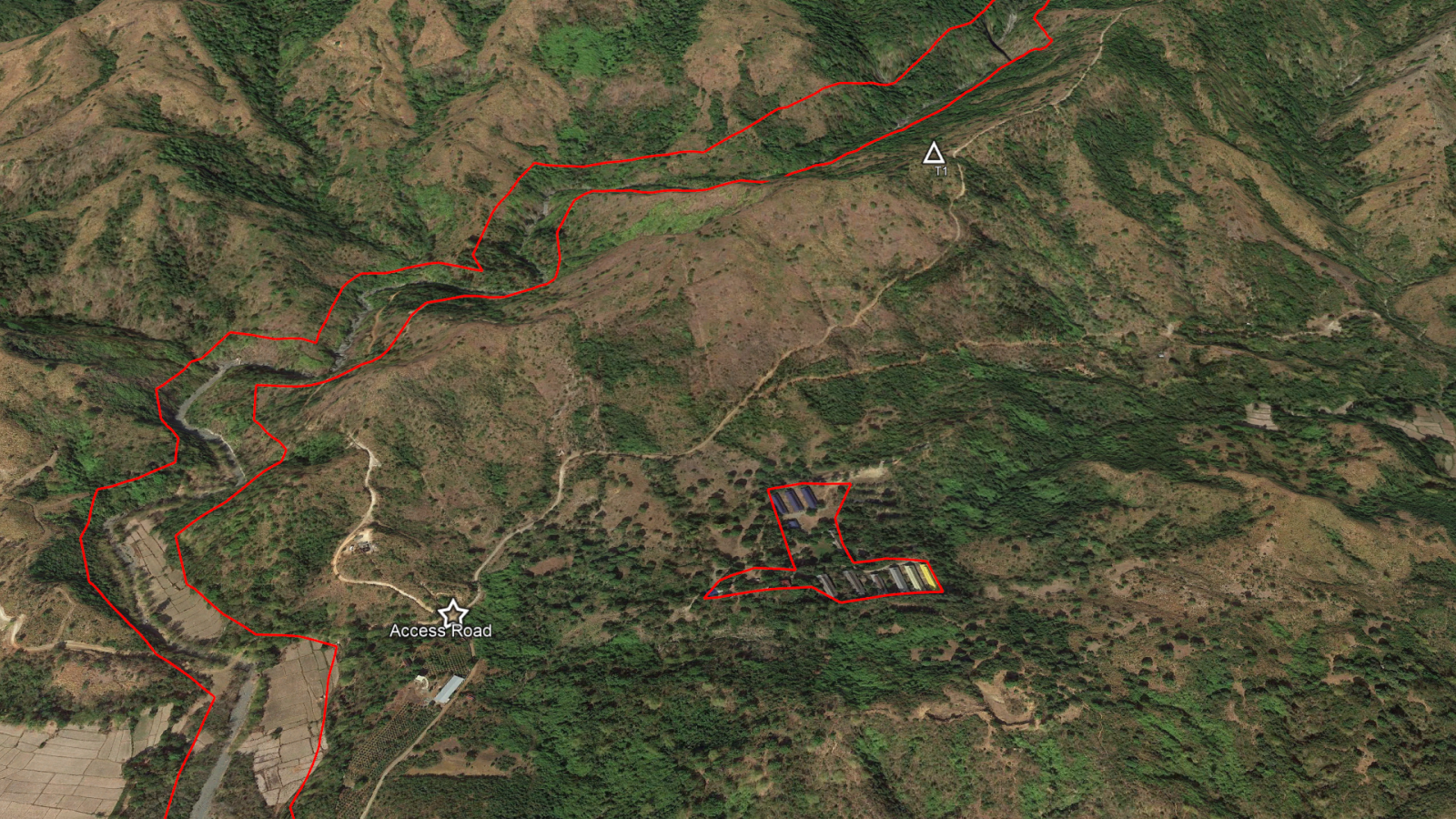The red color polygons are exclusion zones where the road should not go. ##### 1.1 A* Search Basics A* search is an extension of Dijkstra's famous path finding algorithm and is used in many applications to find the shortest path between any two points on a graph. Given two end nodes, the cost of the edges in between are summed and compared in a clever manner. The combination of edges that result in the lowest cost is the shortest / lowest-cost path.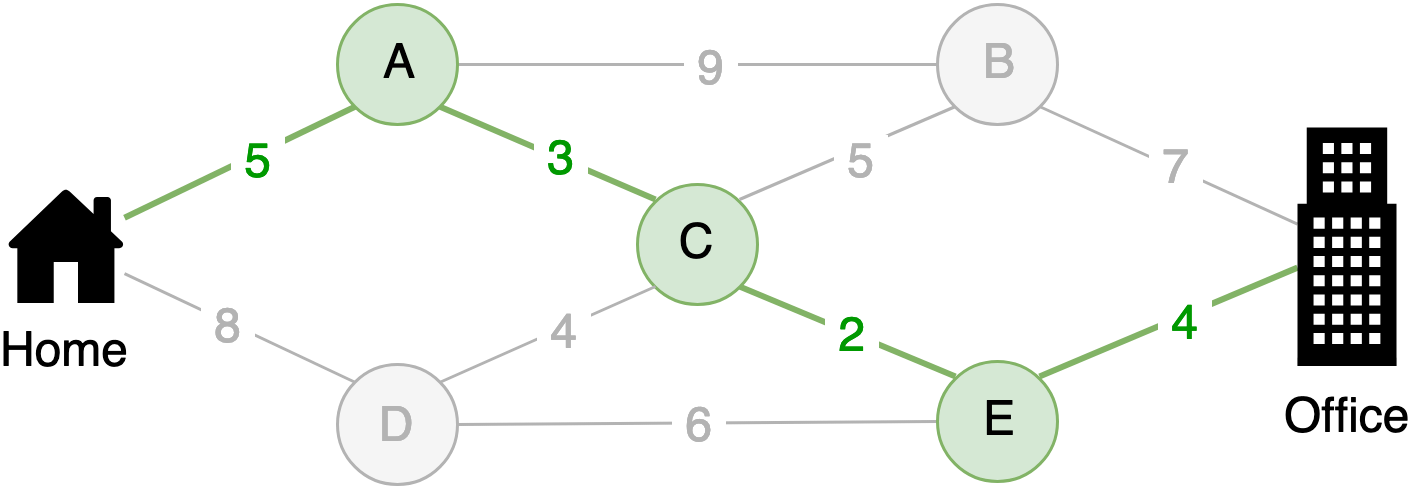The circles are usually called "nodes" and the lines called "edges". Under certain conditions, you can mathematically prove that A* will provide a globally optimal path between two nodes. A detailed explanation of A* can be found here and here. The video below shows how it works in the trivial case of a small maze:A visualization of the A* path-finding algorithm, Wgullyn, CC BY-SA 4.0 via Wikimedia Commons The main attractiveness for using A* is its guarantee to be able to find the lowest cost path with reasonable efficiency (i.e. it won’t take forever). There are many path finding algorithms that are much faster but sacrifice global optima and can only provide local optima. However, A* takes up a very large amount of memory as it needs to keep track of all the nodes it has visited previously in case it needs to backtrack and try new paths. ##### 1.2 A* Search in the Context of Wind Farm Roads The Cost of an Edge In the simplest case, the “cost of an edge” in A* is its distance. However, in the case of wind farm roads, we have to adopt the cost to be a combination of all the following in order of priority: • [rejection criteria] no path shall exceed 14% (8 degrees) but ideally should not exceed 10% (6 degrees) in the longitudinal direction (i.e. the direction of the truck facing the road) • [rejection criteria] the turning radius of paths shall not exceed what the turbine vendor proposes (i.e. around 45m to 75m turning radius for turbines today) • [cost criteria] the lowest total cost (composed of volumetric excavation and road length) • [cost criteria] the lowest amount of earth excavation volume • [cost criteria] the shortest possible distance The rejection criteria are simply edges with infinitely high costs. As for the cost criteria for one edge, it is: $\textnormal{Cost Edge} = \textnormal{Dist} * /m + \textnormal{Excv Vol} * /m^3$ The cost of the whole path is the line integral over the scalar field (elevation, geotechnical condition etc.): $\textnormal{Cost Path} = \int_{path} \textnormal{Cost Edge}\,dl$ The distance is simple but how do we know the excavation area and volume? We take at every 50m intervals, a cross section (perpendicular to the direction of the road) of the elevation and standard benching polygons are drawn to estimate the area of earth required to be cut or filled.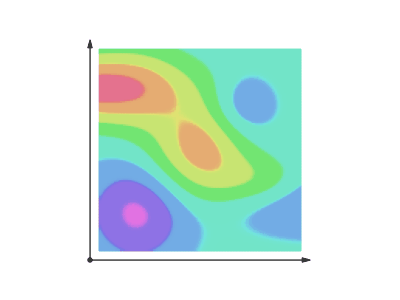When viewing this, assume the line has a thickness (road width). During the benching we can apply concepts such as: • different benching angles for different underlying geotechnical conditions • addition of drainage • addition of inter-array cable trenching • cross streams if any • sealed vs. unsealed roads • road width and road banking for turning (which leads to more excavation as the benching reaches higher up the cross section) • addition of road shoulders for turning clearance • guarantee of vertical profile clearance (e.g. road does not touch belly of truck) By applying these concepts, we get different costs at very high resolution. We then apply the equation above to get each edge’s cost and the A* algorithm evaluates a) which edge is cheaper and b) which combination of edges are cheaper and thereby moves the investigative frontier forward. An example AutoCAD drawing automatically produced by the system is shown below: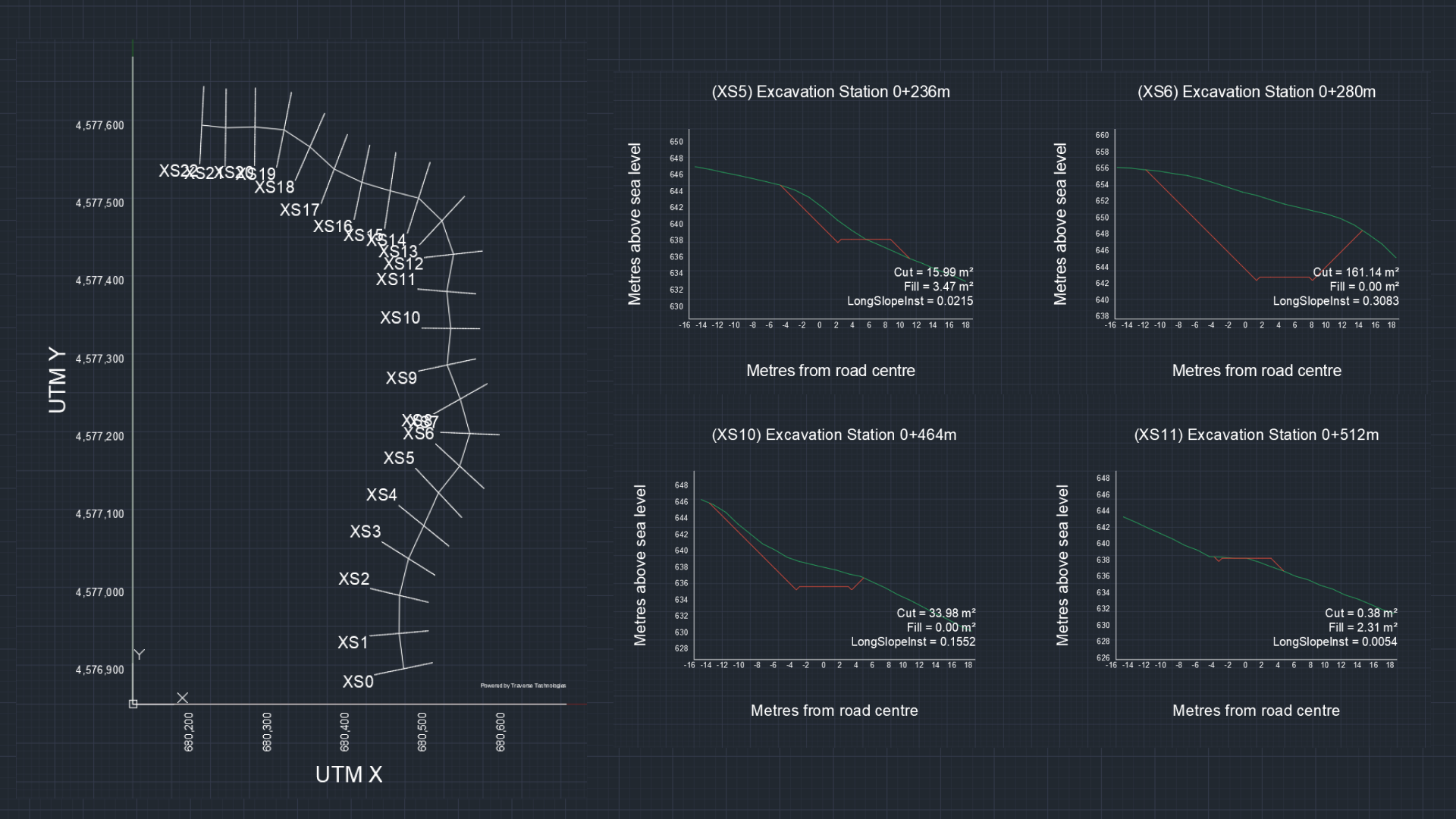AutoCAD drawings produced by the system allows us to audit if there are any bugs both software or civil engineering wise. The “Graph” of a Wind Farm The “graph” of a potential wind farm is simply a regularly sized 2D array like a chess board where every cell has quantitative values such as: • elevation and slope • binary no-go zone (i.e. infinitely costly) • land use classification • geotechnical class if a geotechnical map is available • cost of land acquisition (e.g.$/m2)

The software engineering to manage this graph could get very complicated for large projects or late stage projects that have very high resolution data (such as drone acquired elevation data and geotechnical maps). A* search is computationally intensive and it is preferred if the graph is placed on fast RAM that is closer to the processing cores rather than copied back and forth from a slower hard disk. The largest project we have done to date is a 1.2GW project in India with 218 turbines where the investigative space spanned a 40km by 40km area. Each cell had a 5m resolution which means there are 64,000,000 cells, on top of each of which had 5 quantitative values.

Some of the the strategy we use to manage such large contiguous data is to:

• throw bigger machines at the problem: computers with >200GB of RAM (most laptops only have 8GB)
• “chunk” the whole dataset into bite sized pieces that can be swapped between RAM and hard disk quickly on-the-fly
• efficient choice of compression algorithm that is fast to decompress on-the-fly
##### 1.3 Seeing it in Action

The animation below shows and explains the actual motion of our A* implementation for an actual pair of a turbine point and an access point. This has been slowed down by 20 times. In practice, the whole path-finding takes <1 second for a short distance.

#### 2. The Optimal Paths Between ALL Turbines

Looking at our reference wind farm with 4 turbines and 1 access point (total 5 points), we want to connect all 5 points continuously in most cases. For N turbines there are:

$\textnormal{Ways to Pair All Turbines} = n^{n-2}$

The above formula is called Cayley's formula and the animation below shows all $$5^{5-2} = 125$$ possible pairs for our wind farm. Some of them are of course nonsensical intuitively and can be easily dis-regarded as not a plausible candidate.

We now have to apply A* search (what we described in section 1 of this article, the optimal path between two points) to $$^5C_2$$ = 10 unique pairs. We can then re-use these 10 pairs and apply them on the 125 combinations. The order of combinations and unique pairs grow at a very fast pace as the number of turbines increase:

Turbines Combinations Unique Pairs Brute Force
5 125 10 125
10 1.00E+08 45 1.00E+08
20 2.62E+23 190 2.62E+23
25 1.42E+32 300 1.42E+32
50 3.55E+81 1,225 3.55E+81
100 1.00E+196 4,950 1.00E+196
200 NAN 19,900 NAN

It is not possible in human time to calculate all the combinations due to the double exponent nature of Cayley's formula. We use the concept of Minimum Spanning Trees to reduce this to reasonable calculation times.

##### 2.1 Minimum Spanning Tree Basics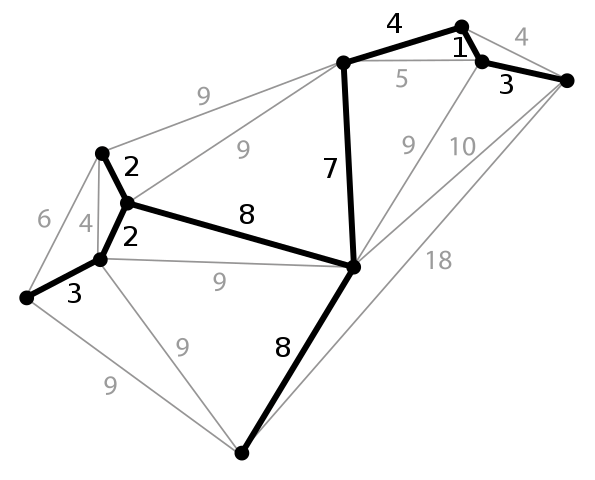The bold lines constitute the Minimum Spanning Tree of this graph. The numbers summed together will result in the lowest value amongst all combinations.

The Minimum Spanning Tree is the set of edge combinations that results in the lowest cost. For example, Prim's algorithm (a type of MST algorithm) is a well-known algorithm that finds this MST in approximately  $$n^2 * \log n$$ time, down from the exponential brute force time of $$n^{n-2}$$. The table below now has an additional column on how many calculations this is reduced by:

Turbines Combinations Unique Pairs Brute Force Prim's Algorithm
5 125 10 125 17
10 1.00E+08 45 1.00E+08 100
20 2.62E+23 190 2.62E+23 520
25 1.42E+32 300 1.42E+32 874
50 3.55E+81 1,225 3.55E+81 4,247
100 1.00E+196 4,950 1.00E+196 20,000
200 NAN 19,900 NAN 92,041

Calculations on the magnitude of 10,000 to 100,000 is manageable with modern hardware.

##### 2.2 Minimum Spanning Tree for Wind Farms

The total number of points in a wind farm is not just the turbines. In addition there are:

• bifurcations or trifurcations (forks)

Existing Roads and Pruning the Forest

Existing roads add hundreds of thousands of points to the forest. At any point in time, we would like to upgrade existing roads for both cost and permitting efficiency. Every point along an existing road is the same as a turbine point. Except that existing road points are an optional connection whereas turbine points are a compulsory connection. Our A* search "weaves" in and out of the existing roads as necessary ensuring that all existing roads fulfill slope and turning radii requirements while also optimizing for low excavation costs.

There are several strategies to reduce the total number of points such as:

• throw more computers: each core works on a single pair so a 100 core computer can process 100 pairs in parallel
• use heuristics: there are some turbines that are way too far from each other even on a beeline basis. Some turbines are at very different elevations from each other and they would never likely connect directly
• human in the loop: force connect a few turbine pairs that intuitively should connect, leave the computer to solve the remaining turbines

Road Forks and The Steiner Tree

In real life, turbines are not always connected directly, we always use road forks. In the image below, we can connect $$ABCD$$ directly. However, the introduction of points $$S_1$$ and $$S_2$$ results in a lower cost tree obviously. In an area like our wind farm, there are an infinite number of candidate points $$S_n$$ that you can add to see which combination of them might reduce the total cost of your tree.

The identification of the best set of points S such that it results in the lowest cost tree is called the Minimum Steiner Tree Problem. Unfortunately this problem is though to be NP-Hard or its NP class is not known, which in ultra simplified terms means it is mathematically provable that no good algorithm exists that can solve the full problem. However, it is a research level topic to find estimations of potential Steiner points. Here at this stage, the WindDesk system uses a completely human in the loop approach where a civil engineer just "litters the landscape" with Forking Point Clouds. They can draw zones of possible forking areas and let the combination of the MST and A* search evaluate the points in these zones to see if they should bifurcate or trifurcate to eventually produce the minimum cost road network.

##### 2.3 Seeing it in Action

When the A* search and MST is put together, this is how it looks like for 4 turbines and an access point:

### 3. Impact on Revenue - Buried Electrical Cables

Because electrical cables are typically buried along the inter-connecting roads (so that they can be dug out and maintained in the future if necessary), optimizing the roads to reduce CAPEX costs inadvertently affects revenue in terms of electrical losses in the inter-array medium voltage cables! Going back to our equation:

$\textnormal{Cost Edge} = \textnormal{Dist} * /m + \textnormal{Excv Vol} * /m^3$

The \$/m cost associated with distance can now be further expanded to not just the physical cost of the cables but the Net Present Value of electrical loss * electricity price. In this way the optimization will attempt to heavily bias towards the shortest possible paths while still fulfilling criteria such as slope and turning radii.

There are multiple other considerations with regards to inter-array electrical cable optimization which we will soon publish in another post.